# Argentometry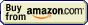## Precipitation titration » Equivalence point calculation

At equivalence point we have just a saturated solution of insoluble salt, so calculation of concentration of the determined ion is identical to the solubility calculations. Just for fun, we can derive seriously looking formula that will describe the concentration.

Let's assume we precipitate salt of a general formula MekXl, with known Ksp:1

Looking at the formula we can see that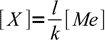2

so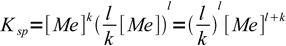3

and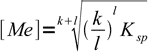4

However, in practice you will probably find it easier to solve the problem manually each time, than to look for formula or trying to remember it. That's what we will do in the following example.

Note, that in the real world it is quite often necessary to account for numerous side reactions - especially for protonation and hydrolysis of both metal cation and ligand.

Example: calculate pZn at the equivalence point of zinc titration with ferrocyanide, assuming pKsp = 16.8.

We don't need any other information about volumes and concentrations! Let's start with dissolution reaction and Ksp:

Zn2Fe(CN)6 → Zn2+ + Fe(CN)64-5

obviously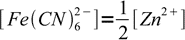6

so7

and8

and finally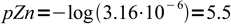9

Let's check, if our general formula gives the same result. From the Zn2Fe(CN)6 formula k=2 and l=1, so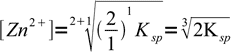10

That's the same.